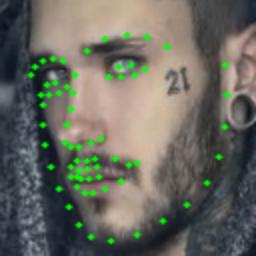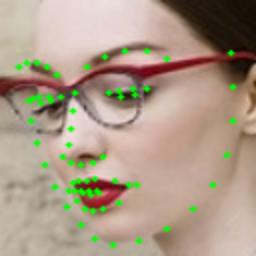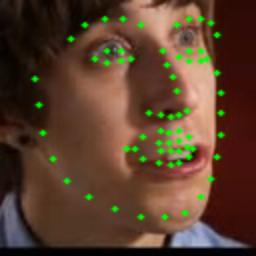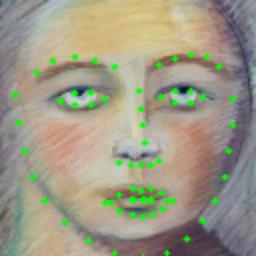# 基于深度学习的人脸特征点检测 - 网络模型构建

## 卷积网络模型结构

Layer nameContentNote
input 128 × 128 × 3 输入层，3通道图像，大小128 × 128像素
conv1 3 × 3 × 32 卷积层，核3×3，stride 1
pool1 2 × 2, stride 2 池化层，Max pooling
conv2 3 × 3 × 64 卷积层，核3×3，stride 1
conv3 3 × 3 × 64 卷积层，核3×3，stride 1
pool2 2 × 2, stride 2 池化层，Max pooling
conv4 3 × 3 × 64 卷积层，核3×3，stride 1
conv5 3 × 3 × 64 卷积层，核3×3，stride 1
pool3 2 × 2, stride 1 池化层，Max pooling
conv6 3 × 3 × 128 卷积层，核3×3，stride 1
conv7 3 × 3 × 128 卷积层，核3×3，stride 1
pool4 2 × 2, stride 1 池化层，Max pooling
conv8 3 × 3 × 256 卷积层，核3×3，stride 1
dense1 1024 全连接层，大小1024
logits 136 输出层，也是全连接层，大小136

## TensorFlow的使用策略

TensorFlow既提供了高层级的API以便让您轻松构建和训练您的模型，也提供了低层级的控制功能以尽可能提高灵活性和性能。

• 定义自己的网络模型结构
• 定义输入函数

### 构建卷积神经网络

#### 卷积层

TensorFlow提供了多种卷积层的实现方法，我推荐采用`tf.layers.conv2d`来实现。这样构建第一个卷积层时可以这样做：

``````conv1 = tf.layers.conv2d(
inputs=inputs,
filters=32,
kernel_size=[3, 3],
strides=(1, 1),
activation=tf.nn.relu)
``````

#### 池化层

``````pool1 = tf.layers.max_pooling2d(
inputs=conv1,
pool_size=[2, 2],
strides=(2, 2),
``````

#### 全连接层

``````logits = tf.layers.dense(
inputs=dense1,
units=136,
activation=None,
use_bias=True,
name="logits")
``````

## 训练结果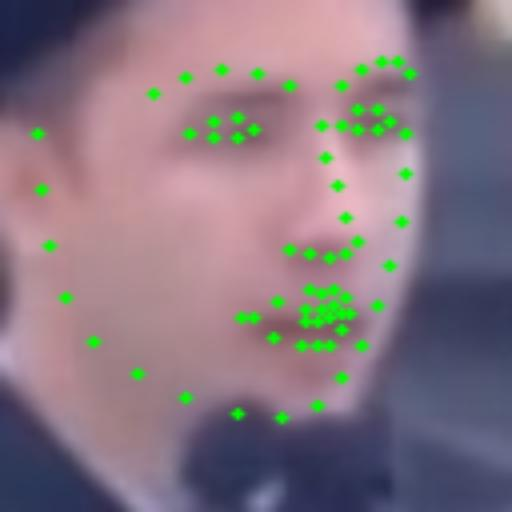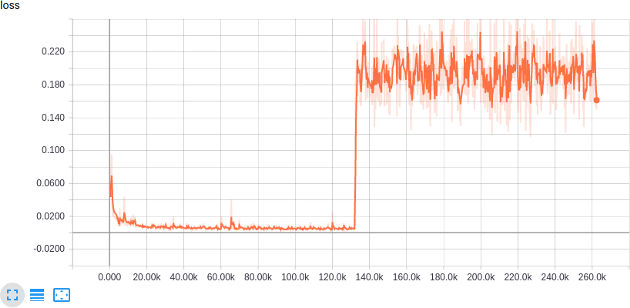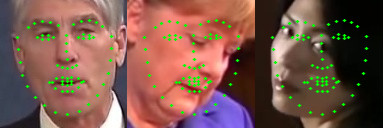### loss函数惹的祸

For example, if labels=[a, b, c] and predictions=[x, y, z], there are three pairs of differences are summed to compute the loss: loss = [ ((a-b) - (x-y)).^2 + ((a-c) - (x-z)).^2 + ((b-c) - (y-z)).^2 ] / 3

### 修正loss函数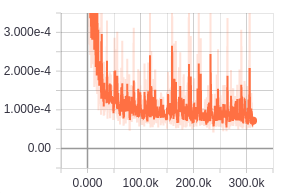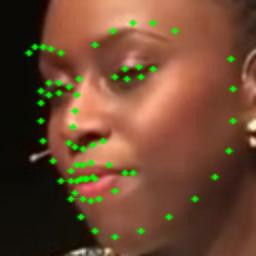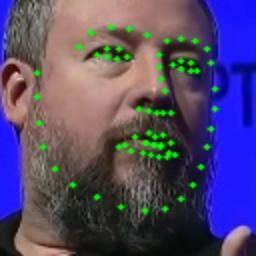### Face in the wild!